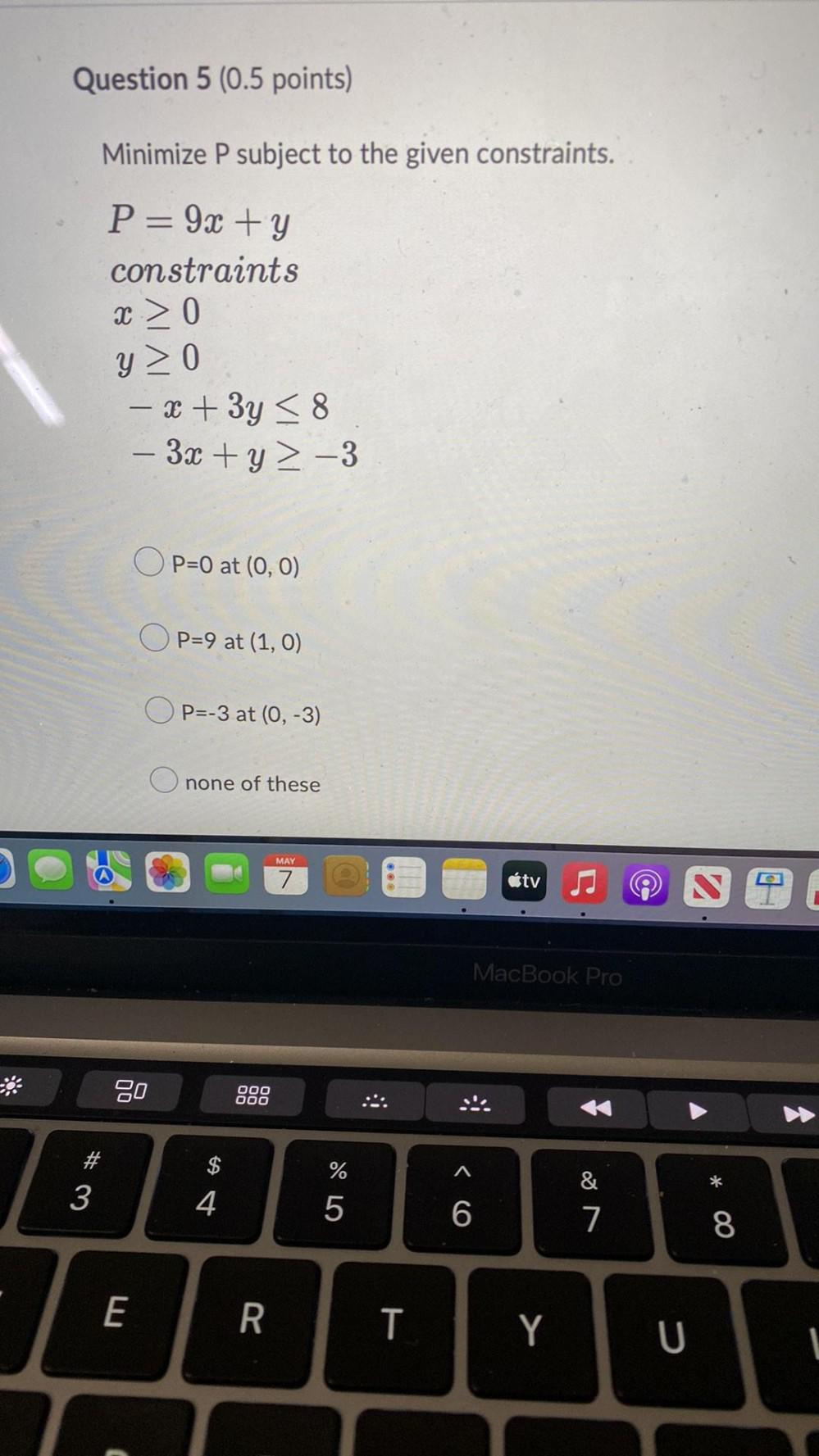Question:

# Minimize P subject to the given constraints. P= 9x + y constraints x ≥ 0 y ≥ 0 -x + 3y ≤ 8 -3x + y ≥ -3 a. P=0 at (0, 0) b. P=9 at (1, 0) c. P=-3 at (0, -3) d. none of theseMinimize P subject to the given constraints. P= 9x + y constraints x ≥ 0 y ≥ 0 -x + 3y ≤ 8 -3x + y ≥ -3 a. P=0 at (0, 0) b. P=9 at (1, 0) c. P=-3 at (0, -3) d. none of these